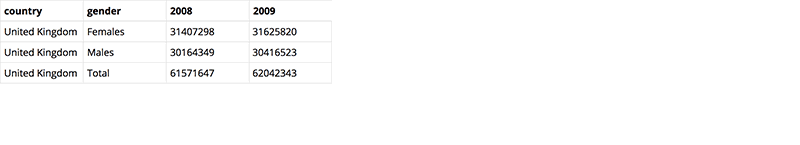Deals Of The Week - hours only!Up to 80% off on all courses and bundles.-Close
Tidyr
Changing data format
8. Gather columns
Summary

## Instruction

We have data for the population of each country. It's divided into three groups: male, female, and total. We have this for each year from 2008 to 2017.

To check the population by year (e.g., for 2008), we need to look at the 2008 column. This data structure is known as wide format. It means that one or more variables (in this case, the year) are in columns rather than rows. Such a format is convenient when calculating a difference in population between years, but it is not ideal. Basic logic in R and tidyverse states that:

• every column is a variable,
• every row is an observation, and
• every cell is a value.

Here, a variable (the year) is not stored as a column. To fix this, we use the gather() function. This function takes data from several or many columns and turns it into key-data pairs of just two columns. In other words, it makes data long instead of wide. Here is how it looks:

amount <- gather(
population, 2008:2017,
key = "year", value = "amount")


This will gather the columns from 2008 to 2017 and put that data (e.g., 2008, 2009, 2010, ..., 2017) into the new year variable. The values associated with each year and country go into the amount column.Inside the gather() function, we have the arguments for the dataset name (population), the range of columns to gather (2008 to 2017), the key argument (which holds the new year column name), and the value argument (which holds the name of the new column that will receive all the values – amount).

## Exercise

We have very similar data about unemployment figures in the unemployment table. This table is also in wide format. Let's fix it.

Use the gather() function to change the format of the data. Use the year columns (2008 to 2017) for gathering. Set year as the key and rate_u (the rate of unemployment) as the value. Assign the result to the unemployment2 variable.

Note: Don't forget to use quotes with the arguments!

### Stuck? Here's a hint!

Type:

unemployment2 <- gather(
unemployment, 2008:2017,
key = "year", value = "rate_u")